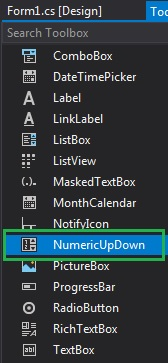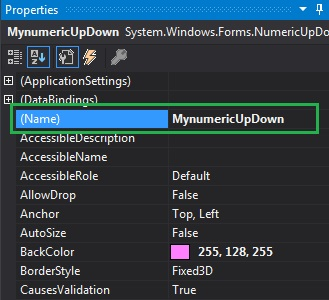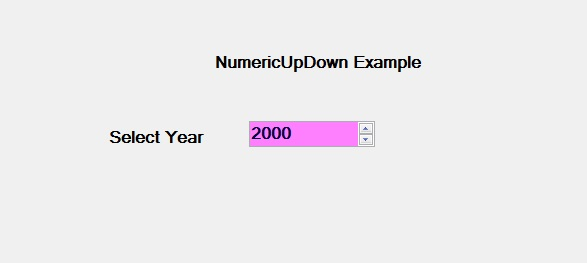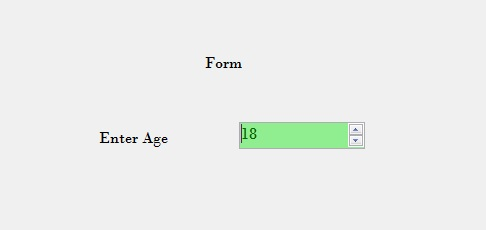# How to set the Name of the NumericUpdown in C#?

• Last Updated : 26 Jul, 2019

In Windows Forms, NumericUpDown control is used to provide a Windows spin box or an up-down control which displays the numeric values. Or in other words, NumericUpDown control provides an interface which moves using up and down arrow and holds some pre-defined numeric value. In NumericUpDown control, you can set the name of the up-down control using the Name Property. You can set this property in two different ways:

1. Design-Time: It is the easiest way to set the name the NumericUpDown as shown in the following steps:

• Step 1: Create a windows form as shown in the below image:
Visual Studio -> File -> New -> Project -> WindowsFormApp• Step 2: Next, drag and drop the NumericUpDown control from the toolbox on the form as shown in the below image:• Step 3: After drag and drop you will go to the properties of the NumericUpDown and set the name of the NumericUpDown as shown in the below image:Output:2. Run-Time: It is a little bit trickier than the above method. In this method, you can set the name of the NumericUpDown control programmatically with the help of given syntax:

`public string Name { get; set; }`

The value of this property is of System.String type, it represents the name of the NumericUpDown. The following steps show how to set name the NumericUpDown dynamically:

• Step 1: Create a NumericUpDown using the NumericUpDown() constructor is provided by the NumericUpDown class.
```// Creating a NumericUpDown
NumericUpDown n = new NumericUpDown();
```
• Step 2: After creating NumericUpDown, set the Name property of the NumericUpDown provided by the NumericUpDown class.
```// Setting the name
n.Name = "MySpinBox";
```
• Step 3: And last add this NumericUpDown control to the form using the following statement:
```// Adding NumericUpDown control on the form
```

Example:

 `using` `System;``using` `System.Collections.Generic;``using` `System.ComponentModel;``using` `System.Data;``using` `System.Drawing;``using` `System.Linq;``using` `System.Text;``using` `System.Threading.Tasks;``using` `System.Windows.Forms;`` ` `namespace` `WindowsFormsApp42 {`` ` `public` `partial` `class` `Form1 : Form {`` ` `    ``public` `Form1()``    ``{``        ``InitializeComponent();``    ``}`` ` `    ``private` `void` `Form1_Load(``object` `sender, EventArgs e)``    ``{``        ``// Creating and setting the ``        ``// properties of the labels``        ``Label l1 = ``new` `Label();``        ``l1.Location = ``new` `Point(348, 61);``        ``l1.Size = ``new` `Size(215, 20);``        ``l1.Text = ``"Form"``;``        ``l1.Font = ``new` `Font(``"Bodoni MT"``, 12);``        ``this``.Controls.Add(l1);`` ` `        ``Label l2 = ``new` `Label();``        ``l2.Location = ``new` `Point(242, 136);``        ``l2.Size = ``new` `Size(103, 20);``        ``l2.Text = ``"Enter Age"``;``        ``l2.Font = ``new` `Font(``"Bodoni MT"``, 12);``        ``this``.Controls.Add(l2);`` ` `        ``// Creating and setting the ``        ``// properties of NumericUpDown``        ``NumericUpDown n = ``new` `NumericUpDown();``        ``n.Location = ``new` `Point(386, 130);``        ``n.Size = ``new` `Size(126, 26);``        ``n.Font = ``new` `Font(``"Bodoni MT"``, 12);``        ``n.Value = 18;``        ``n.Minimum = 18;``        ``n.Maximum = 30;``        ``n.BackColor = Color.LightGreen;``        ``n.ForeColor = Color.DarkGreen;``        ``n.Increment = 1;``        ``n.Name = ``"MySpinBox"``;`` ` `        ``// Adding this control``        ``// to the form``        ``this``.Controls.Add(n);``    ``}``}``}`

Output:My Personal Notes arrow_drop_up